# Inverse function integration formula

## Statement

### In terms of indefinite integrals

Suppose$f$ is a continuous one-one function. Then, we have: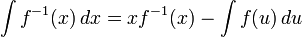$\! \int f^{-1}(x) \, dx = xf^{-1}(x) - \int f(u) \, du$

where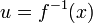$u = f^{-1}(x)$.

More explicitly, if$F$ is an antiderivative for$f$, then: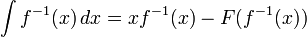$\! \int f^{-1}(x) \, dx = xf^{-1}(x) - F(f^{-1}(x))$

This can be justified either directly or using integration by parts and integration by u-substitution.

### In terms of definite integrals

Suppose$f$ is a continuous one-one function on an interval. Suppose we are integrating$f^{-1}$ on an interval of the form$[a,b]$ that lies in the range of$f$. Then: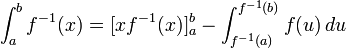$\! \int_a^b f^{-1}(x) = [xf^{-1}(x)]_a^b - \int_{f^{-1}(a)}^{f^{-1}(b)} f(u) \, du$

This simplifies to: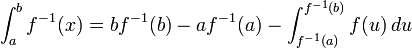$\! \int_a^b f^{-1}(x) = bf^{-1}(b) - af^{-1}(a) - \int_{f^{-1}(a)}^{f^{-1}(b)} f(u) \, du$

More explicitly, if$F$ is an antiderivative for$f$, then:$\! \int_a^b f^{-1}(x) = [xf^{-1}(x)]_a^b - F(f^{-1}(b)) + F(f^{-1}(a))$

## Significance

### Computational feasibility significance

Version type Significance
indefinite integral Given an antiderivative for a continuous one-one function$f$, it is possible to explicitly write down an antiderivative for the inverse function$f^{-1}$ in terms of$f^{-1}$ and the antiderivative for$f$.
definite integral Given an antiderivative for a continuous one-one function$f$, and given knowledge of the values of$f^{-1}$ at$a$ and$b$, it is possible to explicitly compute the definite integral of$f^{-1}$ on$[a,b]$.

## Examples

Original function Domain on which it restricts to a one-one function Inverse function for the restriction to that domain Domain of inverse function (equals range of original function) Antiderivative of original function Antiderivative of inverse function Explanation using inverse function integration formula Alternate explanation using integration by parts
sine function$\sin$$[-\pi/2,\pi/2]$ arc sine function$\arcsin$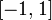$[-1,1]$ negative of cosine function, i.e.,$-\cos$$x \arcsin x + \sqrt{1 - x^2}$ We get$x \arcsin x - (-\cos(\arcsin x)) = x \arcsin x + \cos(\arcsin x)$. Now, use that$\cos$ is nonnegative on the range of$\arcsin$ and that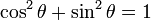$\cos^2\theta + \sin^2\theta = 1$ to rewrite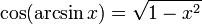$\cos(\arcsin x) = \sqrt{1 - x^2}$. [SHOW MORE]
tangent function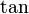$\tan$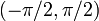$(-\pi/2,\pi/2)$ arc tangent function$\arctan$ all real numbers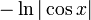$-\ln|\cos x|$, same as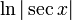$\ln|\sec x|$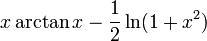$x \arctan x - \frac{1}{2}\ln(1 + x^2)$ We get$x \arctan x - \ln|\sec(\arctan x)|$. Use that$|\sec(\arctan x)| = \sqrt{1 + x^2}$ and simplify. [SHOW MORE]
exponential function$\exp$ all real numbers natural logarithm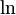$\ln$$(0,\infty)$ exponential function$\exp$$x \ln x - x$ We get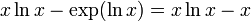$x \ln x - \exp(\ln x) = x \ln x - x$ [SHOW MORE]